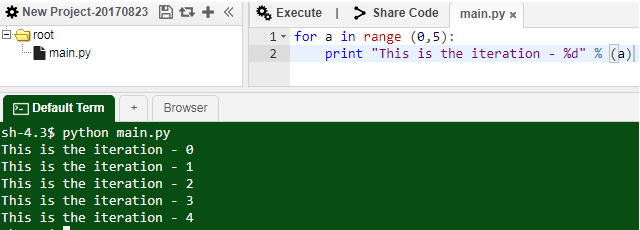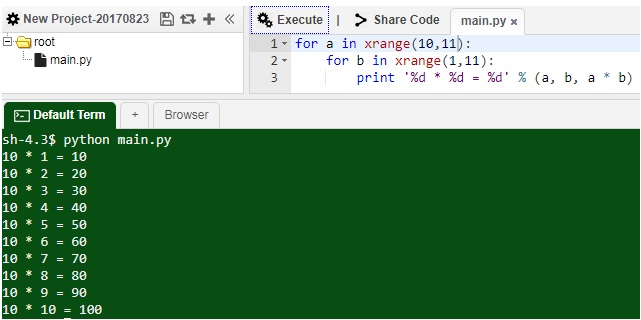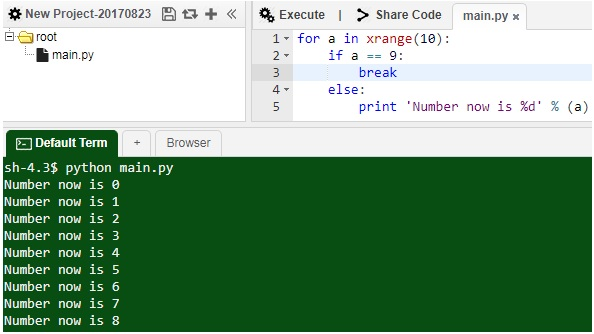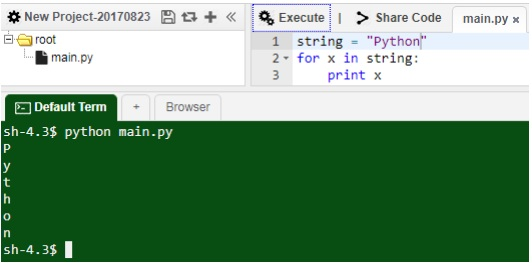# Python for loop

Rating: 4

4187

### Introduction:

Python is a powerful programming language that has started regaining its fame for its usage in Data Science along with the latest technologies like R and etc. Having said that, let us take a look at the tiny winy bits of concepts to get ourselves stronger in this programming language.

In this article, we will try to learn about the loop constructs in the Python programming language. To give you a gist of the loop constructs, there are 2 kinds of it – for and while.

If you want enrich your career and become a professional in python, then visit Mindmajix - a global online training platform : "python online training"   This course will help you to achieve excellence in this domain

## Python for loop

Loops in any traditional programming language (Python, in our case) is used when you need a specific set of code lines to be executed for a specific number of times. There are two kinds of loops in Python – for and while. Python is not going to be any different in this case, so it does the same, iterates over the block of code within the for loop and executes it by the number of times as specified. On the contrary, you would want to check your understanding as against the while loop, which also does the same thing but does it based on a condition to be satisfied or executes it until infinity.

Let us look at the most simple for loop for example:

for a in range (0,5):

print “This is the iteration - %d” % (a)

Output:The above example shows the usage of a basic for loop, which iterates over a print statement for 5 times (starting from 0 to 4, therefore running 5 times). From the example above, it is evident that the for loop construct is meant to be run for a fixed number of times whereas while loop can go on until infinity based on condition.

If you have any programming experience in any other language other than Python as well, you would be able to understand the syntax that has been used for the for loop construct. The variable assignment and the increment of the variable in the Python way. In Python Loops, the variable increment can be controlled in a different manner by generating an appropriate sequence of possible loop values.Methods as like as next() and iter() can be found useful in creating the next possible loop value in Python specifically. This just signifies that you as a programmer won’t be dealing with raw numbers as such for your loop values in Python. Now with this understanding, let us take a step forward and understand how a nested for loop works.

A nested for loop can be easily interpreted as a set of for loops within a for loop. For example, if we want to do a particular thing x number times over an activity that has to be done for every attempt of x, then it is going to loop around x * y times. Not able to understand, take a look at this simple example for you to understand it better.

for a in xrange(10, 11):

for b in xrange(1,11):

print ‘%d * %d = %d’ % (a, b, a * b)

Output:The code above is just trying to print the multiplication table of the number 10, as the outer loop runs only on the loop value of 10 (range is between 10 to 11, starting 10 – so just only one number to deal with and that is 10). The inner loop runs on a range starting 1 to 11 that is 10 numbers, and both these for loops were running on one single of executable code to print the multiplication table of number 10.

Assuming that the concept of nested for loops is understood, let us see how we can exit the for loop without actually having to execute the number of times that it should be using the decision constructs. Take a look at the following example to understand it better:

for an in xrange(10):

if a == 9:

break

else:

print ‘Number now is %d’ % (a)Looking at the code above, it should be clear enough that for every iteration of the for loop, we are checking whether the loop value is equivalent to 9 or not. If it is 9, then we are breaking the loop and coming out and if it is not the case then we are printing the line as shown above.

Now is the time to even understand the other two keywords that we can use in the context of loop constructs in Python – break and continue. The break keyword is used when you want to exit from the loop construct and the continue keyword is used to skip the current block of execution and return back for the next set of execution of the loop construct.

So looking at the previous two examples, you would be under confusion between usage of range and xrange. Let us now clarify this point, so that the next sets of examples make better sense than create any more doubts. range is a Python in-built function that provides us with a sequence of iterable numbers all at once, for the for construct to iterate over.

Python 2.x if considered, range function will return the entire generated sequence of numbers whereas the xrange function provides the numbers generated on demand, only when they are required. The main usage and also the prime difference between these two methods is “resource usage”. Since our examples here are tiny enough to identify the difference, but in practical scenarios this will make much impact. For your information, Python 3.x range function can now be compared with Python 2.x xrange function.

[Related Page: Defining Functions - Python

Let us now take a look at example with string as an iterable.

string = “Python”

for x in string:

print x

Output:Conclusion:

In this article, we have seen what for loops are and why are they used in Python programming language. We have also tried to take a deeper look into the concept with various examples as well.

Hope that you were clear with the concepts after going through this detailed article, please do comment if you have any suggestions to make

If you are interested learn python and build a career in it ? Then checkout our python training near your cities

These courses are incorporated with Live instructor-led training, Industry Use cases, and hands-on live projects. This training program will make you an expert in Microsoft Azure and help you to achieve your dream job.Course Schedule
NameDates
Python TrainingFeb 04 to Feb 19
Python TrainingFeb 07 to Feb 22
Python TrainingFeb 11 to Feb 26
Python TrainingFeb 14 to Mar 01
Last updated: 31 January 2023